Test: Basic Concept - 3

# Test: Basic Concept - 3

Test Description

## 10 Questions MCQ Test | Test: Basic Concept - 3

Test: Basic Concept - 3 for Mechanical Engineering 2023 is part of Mechanical Engineering preparation. The Test: Basic Concept - 3 questions and answers have been prepared according to the Mechanical Engineering exam syllabus.The Test: Basic Concept - 3 MCQs are made for Mechanical Engineering 2023 Exam. Find important definitions, questions, notes, meanings, examples, exercises, MCQs and online tests for Test: Basic Concept - 3 below.
Solutions of Test: Basic Concept - 3 questions in English are available as part of our course for Mechanical Engineering & Test: Basic Concept - 3 solutions in Hindi for Mechanical Engineering course. Download more important topics, notes, lectures and mock test series for Mechanical Engineering Exam by signing up for free. Attempt Test: Basic Concept - 3 | 10 questions in 30 minutes | Mock test for Mechanical Engineering preparation | Free important questions MCQ to study for Mechanical Engineering Exam | Download free PDF with solutions
 1 Crore+ students have signed up on EduRev. Have you?
Test: Basic Concept - 3 - Question 1

### The study of thermodynamics provides answer to the followings: 1. whether a process is feasible or not 2. to quantity the energy required for a process 3. rate or speed with which a process occurs 4. extent to which a reaction/process takes place Which of the above statements are correct?

Test: Basic Concept - 3 - Question 2

### Consider the following statements: 1. Thermodynamic properties are the macroscopic coordinates significant only for systems existing in states of thermodynamic equilibrium. 2. Engineering thermodynamic studies about transfer and transformation of energy. 3. Engineering thermodynamics studies about storage, transfer and transformation of energy. Which of the above is/are correct?

Test: Basic Concept - 3 - Question 3

### An adiabatic boundary is one which

Test: Basic Concept - 3 - Question 4

Match the following List-I with List-ll:

List-I
A. Centrifugal fan
B. Control volume
C. Intensive property
D. Microscopic property

List-ll
1. Open system
2. Internal energy
3. Filling a tire at air station
4. Specific energy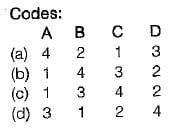Test: Basic Concept - 3 - Question 5

Match the following List-I (Thermometer) with  List -II ( Thermometric property)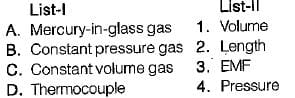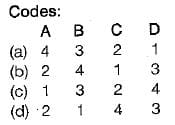Detailed Solution for Test: Basic Concept - 3 - Question 5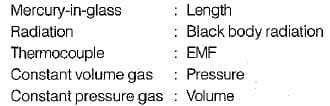Test: Basic Concept - 3 - Question 6

In a quasiequilibrium process, the pressure in a system

Test: Basic Concept - 3 - Question 7

Convert the following readings of pressure to kPa, assuming that the barometer reads 760mm of Hg and match  the List-I and List-II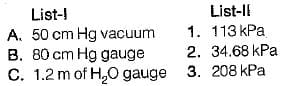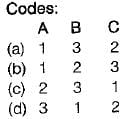Detailed Solution for Test: Basic Concept - 3 - Question 7

50 cm Hg vacuume: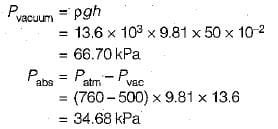80 cm Hg gauge: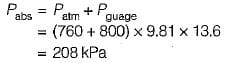1.2 m of H20 guage: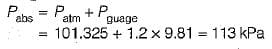Test: Basic Concept - 3 - Question 8

Match the List-I (Terms) with List-ll (Description) and select the correct answer:

List-I
A. Change of state
B. Path
C. Process

List-ll
1. Succession of states
2. One or more properties changes
3. Change of state for specified path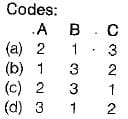Detailed Solution for Test: Basic Concept - 3 - Question 8

Properties are the coordinates to describe the state of a system. They are state variables of the system. Any operation in which one or more of the properties of a system changes is called a change of state. The succession of states passes through during a change of state is called the path of the change of state is called a process. A thermodynamic cycle is defined as a series of state changes such that the final state is identical with the initial state.

Test: Basic Concept - 3 - Question 9

Ice kept in a wail insulated thermo-flask is an example of which system?

Test: Basic Concept - 3 - Question 10

For an isolated system executing a process
1. no heat transfer takes place
2. no work is done
3. no mass crosses the boundary
4. no chemical reaction takes place within the system

Which of the above statement are correct?

Information about Test: Basic Concept - 3 Page
In this test you can find the Exam questions for Test: Basic Concept - 3 solved & explained in the simplest way possible. Besides giving Questions and answers for Test: Basic Concept - 3, EduRev gives you an ample number of Online tests for practice(Scan QR code)